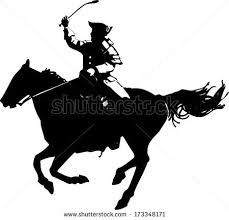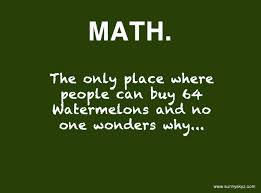•

Team Norton/Prendergast
Check out the supplies you will need for the upcoming 2017-2018 school year

Social Studies
This year we will be covering American History from the 1400's to the last 1700's.
We will also be covering a geography unit early in the year.ScienceScience instruction consists of 3 modules
Levers and Pulleys
Models and Designs
Environment

 Math in Focus   This year you will make connections in math with the Math in Focus program. Math in Focus: The Singapore Approach is the world-class math curriculum from Singapore adapted for U.S. classrooms.    You will learn concepts in an engaging format and practice those concepts to develop a deep understanding through problem solving, games, and discussions.   The Curriculum • building problem-solving skills and strategies • multiplying and dividing 2-digit numbers, fractions, mixed numbers, and decimals • solving equations and evaluating inequalities • finding area of a triangle, and surface area and volume of three-dimensional shapes • using ratios and percents, and finding the probability of an event • applying properties of angles, triangles, and four-sided figures